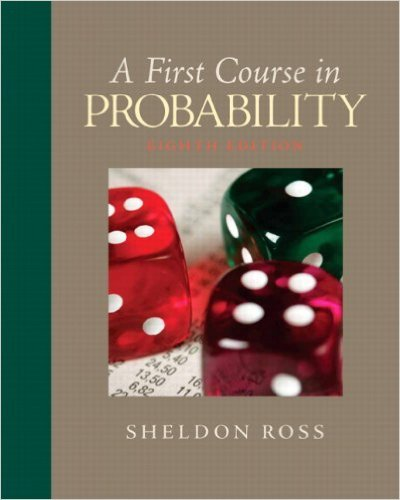×
Get Full Access to First Course In Probability - 8 Edition - Chapter 5 - Problem 5.27
Get Full Access to First Course In Probability - 8 Edition - Chapter 5 - Problem 5.27

×

# In 10,000 independent tosses of a coin, the coinlanded onISBN: 9780136033134 163

## Solution for problem 5.27 Chapter 5

First Course in Probability | 8th Edition

• Textbook Solutions
• 2901 Step-by-step solutions solved by professors and subject experts
• Get 24/7 help from StudySoup virtual teaching assistantsFirst Course in Probability | 8th Edition

4 5 1 344 Reviews
22
0
Problem 5.27

In 10,000 independent tosses of a coin, the coinlanded on heads 5800 times. Is it reasonable toassume that the coin is not fair? Explain.

Step-by-Step Solution:
Step 1 of 3

X, Y Bivariate Random Variables f(u1, 2 ) is density of (X,Y) ∫ ( ) density of X ∫ ( ) density of Y X, Y are independent if f(u1, 2 = f1(u1 2 (u2) If X1, ,2…….X are nndependent Var(X 1 ……..X ) = n + …….. We want to produce to specification “m” “m” = length of a part, content of juice can etc We want to check at the end of the assembly line, if we are meeting the quality of the product (Quality-Control) Process: We choose a sample of size “n” from the product X 1 X 2 …….X n are independent random variables. They have the same distribution. Look at the average of the If X is a random variable then Var(aX) Var( ) = = = ( We

Step 2 of 3

Step 3 of 3

##### ISBN: 9780136033134

First Course in Probability was written by and is associated to the ISBN: 9780136033134. The answer to “In 10,000 independent tosses of a coin, the coinlanded on heads 5800 times. Is it reasonable toassume that the coin is not fair? Explain.” is broken down into a number of easy to follow steps, and 24 words. This full solution covers the following key subjects: coin, independent, explain, fair, heads. This expansive textbook survival guide covers 10 chapters, and 495 solutions. This textbook survival guide was created for the textbook: First Course in Probability, edition: 8. The full step-by-step solution to problem: 5.27 from chapter: 5 was answered by , our top Statistics solution expert on 11/23/17, 05:06AM. Since the solution to 5.27 from 5 chapter was answered, more than 288 students have viewed the full step-by-step answer.

Unlock Textbook Solution